# Level Measurement Instrumentation Questions Answers## Level Measurement Instrumentation Questions Answers

Q1. What is the liquid level in the tank ?

(Circle the correct option)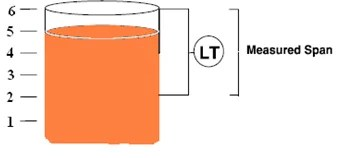1. 75% of measured span
2. 83% of measured span
3. 50 % of measured span
4. 25% of measured span

Q2. In displacement type level measurement, which law is used ?

1. Charles law
2. Ohms law
4. Archimedes principle

Q3. The weight of the displacer tube is  25 pounds. The buoyant force is 16 pounds when the displacer is  submerged completely. What will be apparent weight of the displacer when submerged to 25% of water level?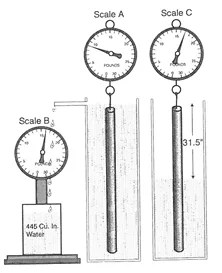1. 21 pounds
2. 17 pounds
3. 25 pounds
4. 16 pounds

Q4. Under what circumstances would it be most appropriate to use dry leg system to measure the level in a closed vessel? (Circle the correct option)

1. Gas above the liquid condenses easily.
2. Gas above the liquid cannot condense.
3. Whenever a DP transmitter is used
4. Only when a Gauge Pressure Transmitter

Q5. In a wet leg system, the LP impulse line is completely filled with a liquid. This is

1. Just to create a negative pressure
2. To compensate atmospheric pressure
3. To avoid measurement error when the gas above the liquid condenses

Q6. In an HTG system, density cannot be calculated if the product volume is _________ the middle transmitter.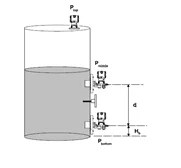1. Above
2. Below
3. In level with

Q7.  Specific  gravity of a material  is the ratio of density of a material to the density of

_______Water_____

Q8. The boundary between the immiscible liquids is called

_______Interface_________.

Q9.In the interface level measurement using DP transmitter the  assumptions are that  at lower calibrated value the(4 mA point) , the tank is filled with ________ fluid  ( heavier/lighter ) and at the upper calibrated value  the tank is filled with ________________ fluid ( heavier/lighter).

Q10. In ultrasonic level measurement, if the round trip time  is t, the distance

D=  Velocity of _______Sound_____× t/2

Q11. A displacer level transmitter has a 30 inch tube and is used to measure the level of liquid with a specific gravity of 0.9. You need to calibrate the transmitter using sweet water. Calculate the  depth of sweet water in which the displacer must be immersed to provide a maximum range  output (20 mA) from the transmitter.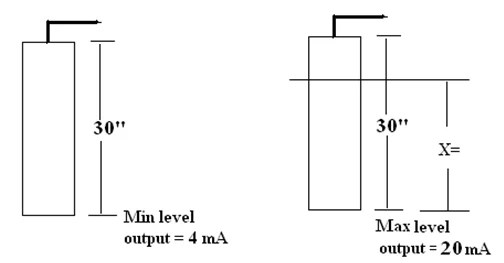Ans:  P = H * D

= 30 * 0.9

=  27 In

Q12. How do you do a zero check on a DP transmitter using 3 valve manifold ?

Give Answer: Or check in Comment

Q13. In radar transmitter, The amount of reflected energy from the fluid surface is dependent on its dielectric constant. Is this statement True or False ?

Give Answer or See in comment.

Q14. Can we use capacitor type level measurement  if the process material is  conducting ?

Yes

Q15. Calculate the calibrated points for zero and span in inches of H2O.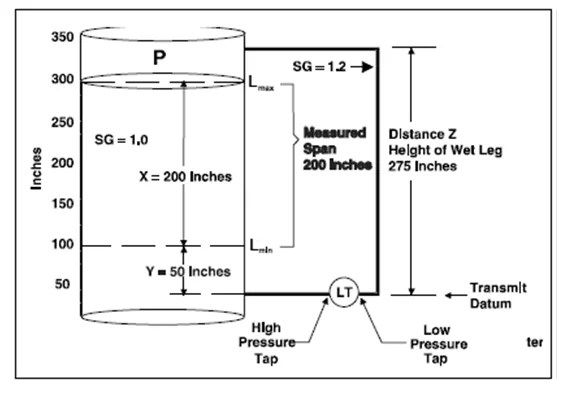4 mA  =  Y*SG – Z*SG

=   50*1.0 – 275*1.2

=  50-330

= -280 in H2O

20 mA  =  (X+Y)*SG – Z*SG

=   250*1.0  –  275*1.2

=     250 – 330

=    -80 in H2O

RANGE    -80 to -280 in H2O

Following videos related to Level Instrumentation and sole property of PAKTECHPOINT and enjoy gaining the knowledge and share to others.

paktechpoint.com

#### You may also like...

error: tected !!Content is pro